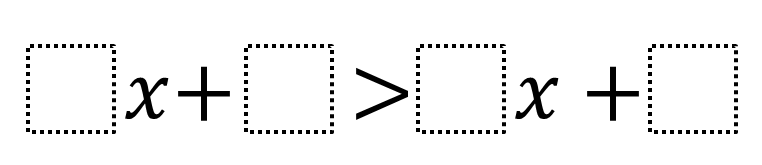# Create an Inequality, Given a Solution Set

Directions: Using the digits 0 to 9 at most one time each, fill in the boxes to create an inequality whose solution set is x < -1/2.### Hint

What does it mean to have a solution set in an inequality? How can you use the solution set “border number” to help you write an inequality?

There are many correct answers. Some possible responses could be:
2x + 5 > 4x + 6;
1x + 5 > 7x + 8;
9x + 6 < 5x + 4

Source: Daniel Luevanos

## Difference of Squares and Sum of Cubes

Directions: Using the digits 0 to 9 at most one time each, place a digit …

1.I love this question. I’ve been wondering what to call the “border number.” I’ve been calling it a “limit.” What are your thoughts on that?

•Glad you like the question, Lane! I usually refer to the “border number” or “limit” as the “boundary point.” I never thought about using “limit” as part of my discussion with students, but now I’m going to. Thanks!

2.My students also came up with these solutions:

1x + 4 > 3x + 5
1x + 5 > 3x + 6
1x + 7 > 3x + 8
1x + 8 > 3x + 9
5x + 8 > 7x + 9
2x + 5 > 4x + 6
4x + 8 > 6x + 9

•These are all great solutions! Thank you for sharing!

•Oh wow 😊😊😊😊 your students are bright !!!!!!!!!!!!!!!!!. Thanks for building the children of the nation

3.Thank you very much for the help to develop us students by helping us some difficult questions we can´t manage. May God bless you

4.Thank you very much for the problem!

I have a student that developed an equation to represent the situation as long as no number is repeated, where x is any number between 1 and 9.

x + (constant) > x +1 + (constant + 2)